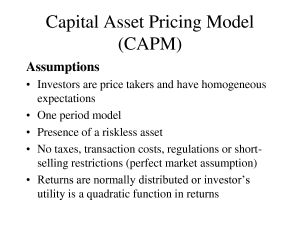# Capital Asset Pricing Model (CAPM)

Certify and Increase Opportunity.
Be
Govt. Certified -Equity Research Analyst

## The Capital Asset Pricing Model (CAPM)

CAPM states the price of a stock is tied to two variables:  the time value of money, and the risk of the stock itself.  When we look at some of the formulas used in the CAPM later on, we’ll see that the time value of money is represented by the risk-free rate of interest or rf.

When measuring the risk of the stock itself, the capital asset pricing model explains that risk in terms that are relative to the overall stock market risk. Fortunately, this is exactly what a stock’s beta measures.

To figure out the expected rate of return of a particular stock, the CAPM formula only requires three variables:

rf = which is equal to the risk-free rate of an investment
rm = which is equal to the overall stock market risk
B = which is equal to the stock’s beta
Using CAPM to Calculate Stock ReturnsWe’ve already discussed in our publication, Calculating Stock Prices, how the price of a common stock is equal to the discounted value of the expected dividend stream and the end-of-period stock price. The CAPM helps investors to figure out the expected return on a particular investment.

### Apply for Equity Research Certification Now!!

http://www.vskills.in/certification/Certified-Equity-Research-Analyst

### Get industry recognized certification – Contact us